JEE  >  Part Test - 15 (JEE Advanced)

# Part Test - 15 (JEE Advanced)

Test Description

## 60 Questions MCQ Test National Level Test Series for JEE Advanced 2020 | Part Test - 15 (JEE Advanced)

Part Test - 15 (JEE Advanced) for JEE 2023 is part of National Level Test Series for JEE Advanced 2020 preparation. The Part Test - 15 (JEE Advanced) questions and answers have been prepared according to the JEE exam syllabus.The Part Test - 15 (JEE Advanced) MCQs are made for JEE 2023 Exam. Find important definitions, questions, notes, meanings, examples, exercises, MCQs and online tests for Part Test - 15 (JEE Advanced) below.
 1 Crore+ students have signed up on EduRev. Have you?
Part Test - 15 (JEE Advanced) - Question 1

### Two identical conducting balls A and B have positive charges q1 and q2 respectively. But q1 ≠ q2. The balls are brought together so that they touch each other and then kept in their original positions. The force between them is ______.

Part Test - 15 (JEE Advanced) - Question 2

### How many 6mF, 200 V condensers are needed to make a condenser of 18 pF, 600 V?

Part Test - 15 (JEE Advanced) - Question 3

### A closed organ pipe and an open organ pipe of same length produce 2 beats/second while vibrating in their fundamental modes. The length of the open organ pipe is halved and that of closed pipe is doubled. Then, the number of beats produced per second while vibrating in the fundamental mode is ______.

Part Test - 15 (JEE Advanced) - Question 4

A current of 5A is flowing at 220 V in the primary coil of a transformer. If the voltage produced in the secondary coil is 2200 V and 50% of power is lost, then the current in the secondary will be

Part Test - 15 (JEE Advanced) - Question 5

A convex lens of focal length 30 cm produces 5 times magnified real image of an object. What is the object distance?

Part Test - 15 (JEE Advanced) - Question 6

In a series resonant R-L-C circuit, the voltage across R is 100 V and the value of R = 1000 W. The capacitance of the capacitor is 2 ´ 10-6 F; angular frequency of AC is 200 rad s-1. Then the P.D. across the inductance coil is ______.

Part Test - 15 (JEE Advanced) - Question 7

There is a uniform magnetic field directed perpendicular and into the plane of the paper. An irregular shaped conducting loop is slowly changing into a circular loop in the plane of the paper. Then ______.

Part Test - 15 (JEE Advanced) - Question 8

Two equal masses hang on either side of a pulley at the same height from the ground. The mass on the right is given a horizontal speed, after some time.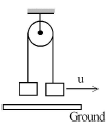Detailed Solution for Part Test - 15 (JEE Advanced) - Question 8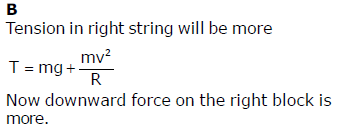Part Test - 15 (JEE Advanced) - Question 9

A metallic surface is irradiated with monochromatic light of variable wavelength. Above a wavelength of 5000 Å, no photoelectrons are emitted from the surface. With an unknown wavelength, a stopping potential of 3V is necessary to eliminate the photo current. The unknown wavelength is

Detailed Solution for Part Test - 15 (JEE Advanced) - Question 9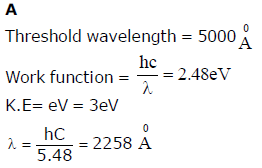Part Test - 15 (JEE Advanced) - Question 10

Three liquids of equal masses are taken in three identical cubical vessels A, B and C. Their densities are PA, PB and PC respectively. But PA < PB < PC. The force exerted by the liquid on the base of the cubical vessel is ______

Part Test - 15 (JEE Advanced) - Question 11

Two identical conducting balls A and B have positive charges q1 and q2 respectively. But q1 ≠ q2. The balls are brought together so that they touch each other and then kept in their original positions. The force between them is ______.

Part Test - 15 (JEE Advanced) - Question 12

Two tangent galvanometers A and B are identical except in their number of turns. They are connected in series. On passing a current through them, deflections of 600 and 300 are produced. The ratio of the number of turns in A and B is

Part Test - 15 (JEE Advanced) - Question 13

Water is in streamline flow along a horizontal pipe with nonuniform cross-section. At a point in the pipe where the area of cross-section is 10 cm2, the velocity of water is 1 ms-1 and the pressure is 2000 Pa. The pressure at another point where the cross-sectional area is 5 cm2 is ______.

*Multiple options can be correct
Part Test - 15 (JEE Advanced) - Question 14

A vertical capillary tube with inside radius 0.25 mm is submerged into water so that the length of its part over the water surface is equal to 25 mm. Surface tension of water is 73x10-3 N/m and angle of contact is zero degree for glass and water, acceleration due to gravity is 9.8 m/s2. Then choose correct statement.

Where R is radius of meniscus and h is height of water in capillary tube.

Detailed Solution for Part Test - 15 (JEE Advanced) - Question 14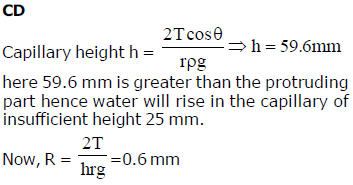*Multiple options can be correct
Part Test - 15 (JEE Advanced) - Question 15

Ideal fluid flows along a atube of uniform cross section, located in a horizontal plane and bent as shown in figure. The flow is steady, 1 and 2 are two points in the tube. If  P1 and P2 are pressure at the two points and v1 and v2 are the respective velocities, given that point 1 and 2 are at nearly same vertical level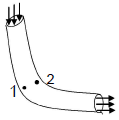Detailed Solution for Part Test - 15 (JEE Advanced) - Question 15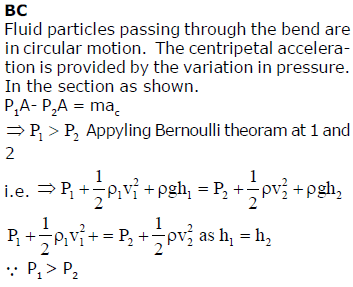*Answer can only contain numeric values
Part Test - 15 (JEE Advanced) - Question 16

Four wires of equal length 2m are arranged as shown in the figure. Wires 2, 3 and 4 are of equal cross sectional area and wire 1 is of half the cross section of these wires. By how much distance pointer at point 5 must be moved to get null point in cm ?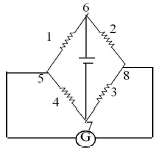Detailed Solution for Part Test - 15 (JEE Advanced) - Question 16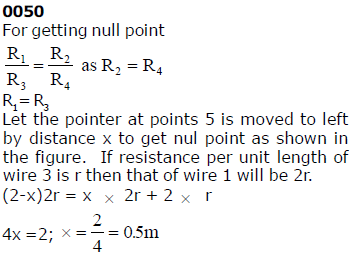*Answer can only contain numeric values
Part Test - 15 (JEE Advanced) - Question 17

For the arrangement of the potentiometer shown in the figure, the balance point is obtained at a distance 75 cm from A when the key k is open.The second balance point is obtained at 60 cm from A when the key  k is closed. Find the internal resistance (in ?) of the battery E1.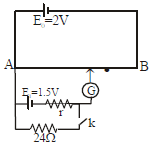Detailed Solution for Part Test - 15 (JEE Advanced) - Question 17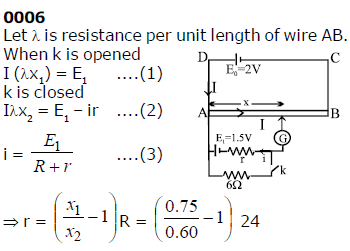*Answer can only contain numeric values
Part Test - 15 (JEE Advanced) - Question 18

A cylinder fitted with a spring loaded piston shown in figure contains 0.01m3 gas at a pressure of 105 Pa. The cross-sectional area of the piston is 0.05 m2. Initially the spring does not touch the piston but atmospheric pressure of 105 Pa acts on the piston. The gas is slowly heated till the volume is increased to three times the original value. If the force constant of  the spring is 25 kN / m, calculate the work done (in Joule) by the gas.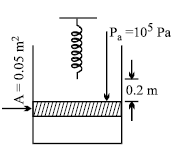Detailed Solution for Part Test - 15 (JEE Advanced) - Question 18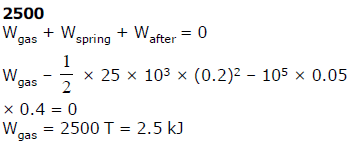*Answer can only contain numeric values
Part Test - 15 (JEE Advanced) - Question 19

The intensity received at the focus of the lens is I when no glass slab has been placed in front of the slit. Both the slits are of the same dimension and the plane wavefront incident perpendicularly on them, has wavelength λ. On placing the glass slab, the intensity reduces to 3I/4 at the focus. Find out the minimum thickness of the glass slab (in Å) if its refractive index is 3/2. Given λ = 6933Å, µ = 1.5.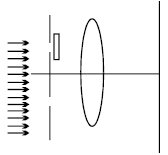Detailed Solution for Part Test - 15 (JEE Advanced) - Question 19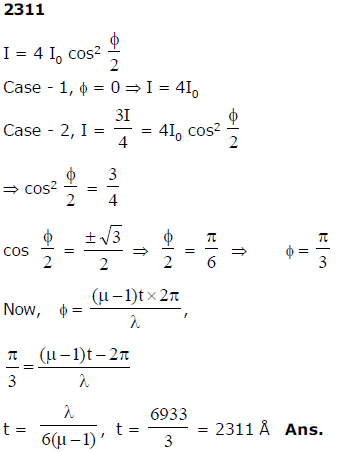*Answer can only contain numeric values
Part Test - 15 (JEE Advanced) - Question 20

A rod of length R and mass M is free to rotate about a horizontal axis passing through hinge P as shown in the figure. First it is taken aside such that it becomes horizontal and then released. At the lowest point the rod hits the block B of mass m and stops. If mass of rod is (√60) kg, find mass of the block if it just complete the circle.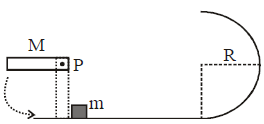Detailed Solution for Part Test - 15 (JEE Advanced) - Question 20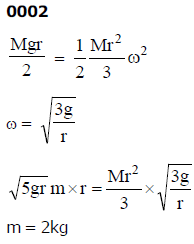Part Test - 15 (JEE Advanced) - Question 21

Which among the following factors is the most important in making fluorine the strongest oxidizing halogen

Detailed Solution for Part Test - 15 (JEE Advanced) - Question 21

Hydration energy

Part Test - 15 (JEE Advanced) - Question 22

The standard electrode potential for the half cell reactions are:

Zn++ + 2e →  Zn E° = – 0.76 V

Fe++ + 2e → Fe E° = – 0.44 V

The E.M.F. of the cell reaction :

Fe++ + Zn →  Zn++ +Fe is

Part Test - 15 (JEE Advanced) - Question 23

An elemental crystal has a density of 8570 kg m–3. The packing efficiency is 0.68. If the closest distance between neighbouring atoms is 2.86 Å, the mass of one atom is(1 amu=1.66×10–27 kg)

Detailed Solution for Part Test - 15 (JEE Advanced) - Question 23

93 amu

Part Test - 15 (JEE Advanced) - Question 24

The value of DH for the reaction

X2g + 4Y2(g) Ý 2XY4(g) is less than zero. Formation of XY4(g) will be favoured at

Part Test - 15 (JEE Advanced) - Question 25

The stabilization of a dispersed phase in a lyophobic colloid is due to:

Detailed Solution for Part Test - 15 (JEE Advanced) - Question 25

the formation of an electrical  layer between two phases.

Part Test - 15 (JEE Advanced) - Question 26

Which of the following used is a promoter in Haber’s method to manufacture of ammonia

Detailed Solution for Part Test - 15 (JEE Advanced) - Question 26

Mo

Part Test - 15 (JEE Advanced) - Question 27

Calculate no. of stereogenic center in following molecule.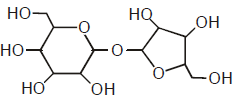Detailed Solution for Part Test - 15 (JEE Advanced) - Question 27

D

Ans is 9

Part Test - 15 (JEE Advanced) - Question 28

The ease of liquification and polarizibility of noble gases decreases in the order

Detailed Solution for Part Test - 15 (JEE Advanced) - Question 28

He<Ne<Ar<Kr<Xe

Part Test - 15 (JEE Advanced) - Question 29

For a reversible reaction : X(g) + 3Y(g) ⇌ 2Z(g)DH = –40 kJ the standard entropies of X, Y and Z arc 60, 40 and 50 JKmolrespectively.The temperature at which the above reaction attains equilibrium is about

Part Test - 15 (JEE Advanced) - Question 30

The rate constant, the activation energy, and the Arrhenius parameter of a chemical reaction at 25°C are 3.0×10–4 s–1 104.4 kJ mol–1, and 6.0 × 1014 s–1, respectively. The value of the rate constant as T → ∞ is

Detailed Solution for Part Test - 15 (JEE Advanced) - Question 30

6.0×1014 s–1

*Multiple options can be correct
Part Test - 15 (JEE Advanced) - Question 31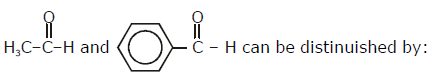Detailed Solution for Part Test - 15 (JEE Advanced) - Question 31

B,C

*Multiple options can be correct
Part Test - 15 (JEE Advanced) - Question 32

Peroxodisulphate salts (e.g., Na2S2O8) are strong oxidizing agents used as bleaching agents for fats, oils etc.
Given: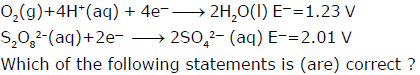Detailed Solution for Part Test - 15 (JEE Advanced) - Question 32

C,D

Part Test - 15 (JEE Advanced) - Question 33

For the following reaction C6H12O6(aq) + H2(g) ⇌ C6H14O6(aq)Which one of the following is not affected by the addition of catalyst?

*Multiple options can be correct
Part Test - 15 (JEE Advanced) - Question 34

Which of the following is/are paramagnetic ?

Detailed Solution for Part Test - 15 (JEE Advanced) - Question 34

[Fe(NH3)6]2+

Part Test - 15 (JEE Advanced) - Question 35

Which of the following is correct option for free expansion of an ideal gas under adiabatic condition?

*Answer can only contain numeric values
Part Test - 15 (JEE Advanced) - Question 36

The number of 3C–2e bond present in a diborane molecular are:

Detailed Solution for Part Test - 15 (JEE Advanced) - Question 36

0002

*Answer can only contain numeric values
Part Test - 15 (JEE Advanced) - Question 37

The freezing point of a 0.08 molal aqueous solution of NaHSO4 is – 0.372ºC. Calculate the % dissociation for the reaction at the given temperature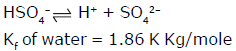Detailed Solution for Part Test - 15 (JEE Advanced) - Question 37

0050

*Answer can only contain numeric values
Part Test - 15 (JEE Advanced) - Question 38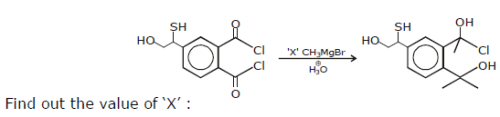Detailed Solution for Part Test - 15 (JEE Advanced) - Question 38

0006

*Answer can only contain numeric values
Part Test - 15 (JEE Advanced) - Question 39

50 ml of 0.05 M Na2CO3 is titrated against 0.1 M HCl. On adding 40 ml of HCl. pH of the solution will be:

[Given : For H2CO3 :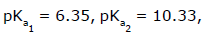, log3 = 0.477, log 2 = 0.3 ]

Detailed Solution for Part Test - 15 (JEE Advanced) - Question 39

0006

*Answer can only contain numeric values
Part Test - 15 (JEE Advanced) - Question 40

Of the following amines how many can give carbyl amine reaction.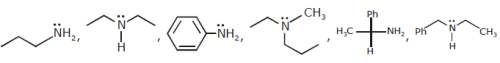Detailed Solution for Part Test - 15 (JEE Advanced) - Question 40

0003

Part Test - 15 (JEE Advanced) - Question 41

The number of positive integer that divides 101005 but not 101004 is

Detailed Solution for Part Test - 15 (JEE Advanced) - Question 41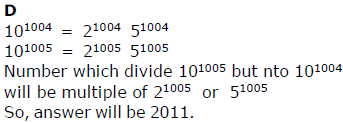Part Test - 15 (JEE Advanced) - Question 42

The sides of a triangle are the roots of the cubic equation x3 – 13x2 + 54x – 72 = 0 then the area of the triangle in square units is

Detailed Solution for Part Test - 15 (JEE Advanced) - Question 42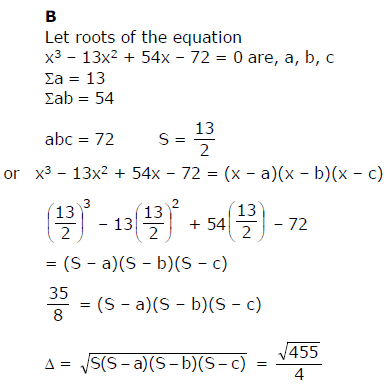Part Test - 15 (JEE Advanced) - Question 43

The function f(x) = ax2 – c satisfies
–4 < f(1) < –1 and –1 < f(2) < 5. Which of the following statements is true ?

Detailed Solution for Part Test - 15 (JEE Advanced) - Question 43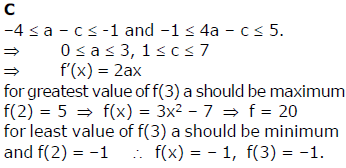Part Test - 15 (JEE Advanced) - Question 44

Which one of the following is present as an active ingredient in bleaching powder for bleaching action?

Part Test - 15 (JEE Advanced) - Question 45

When sulphur dioxide is passed in an acidified K2CrO7 solution, the oxidation state of sulphur is changed from

Part Test - 15 (JEE Advanced) - Question 46

Arrange the following in the increasing order of their basic strengths :

CH3NH2, (CH3)2NH, (CH3)3N, NH3

Part Test - 15 (JEE Advanced) - Question 47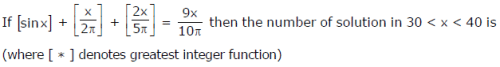Detailed Solution for Part Test - 15 (JEE Advanced) - Question 47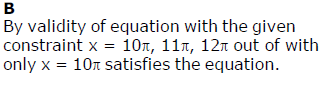Part Test - 15 (JEE Advanced) - Question 48

An ellipse E: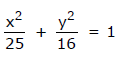and hyperbola ‘H’ whose length of transverse axis is equal to length of semi minor axis if ellipse E; are confocal. Point ‘P’ is the common point for E and H. If M1 and M2 are foot of perpendicular from point ‘P’ on the corresponding directrix of E and H respectively then (M1M2) is

Detailed Solution for Part Test - 15 (JEE Advanced) - Question 48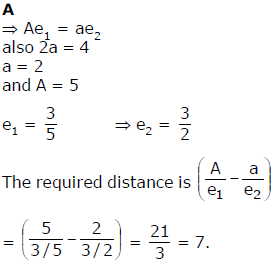Part Test - 15 (JEE Advanced) - Question 49

The typical range of molar enthalpies for the strongest intermolecular (Hydrogen) bonds is

Part Test - 15 (JEE Advanced) - Question 50

The ordered pair (a, b) such that x2 – x – 1 divides the polynomial ax9 + bx8 + 1 is

Detailed Solution for Part Test - 15 (JEE Advanced) - Question 50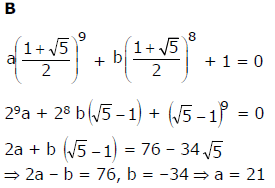*Multiple options can be correct
Part Test - 15 (JEE Advanced) - Question 51

The co-ordinates of the vertices A, B, C of a triangle ABC whose ortho centre is H(–3, 10) and circumcentre is O(–2, –3) and mid point of BC is D (1, 3) are

Detailed Solution for Part Test - 15 (JEE Advanced) - Question 51

A, B & D Are correct Answers

Part Test - 15 (JEE Advanced) - Question 52

The amount of heat evolved when 500 cm3 of 0.1 M HCI is mixed with 200 cm3of 0.2 M NaOH is ______.

Part Test - 15 (JEE Advanced) - Question 53

Standard electrode potential for Sn4+ / Sn2+ couple is +0.15 V and that for the Cr3+ / Cr couple is –0.74 V. These two couples in their standard state are connected to make a cell. The cell potential will be

Part Test - 15 (JEE Advanced) - Question 54

Three liquids of equal masses are taken in three identical cubical vessels A, B and C. Their densities are PA, PB and PC respectively. But PA < PB < PC. The force exerted by the liquid on the base of the cubical vessel is ______

Part Test - 15 (JEE Advanced) - Question 55

Two steel wires of he same radius have their lengths in the ratio of 1:2. if they are stretched by the same force, then the strains produced in the two wires will be in the ratio of

*Answer can only contain numeric values
Part Test - 15 (JEE Advanced) - Question 56

Find the numbers of points of intersection of curve sin x = cos y and circle x2 + y2 = 1.

Detailed Solution for Part Test - 15 (JEE Advanced) - Question 56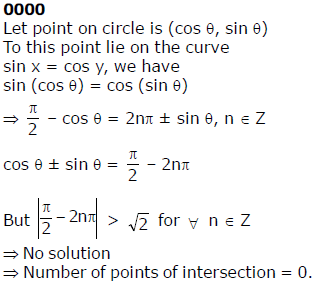*Answer can only contain numeric values
Part Test - 15 (JEE Advanced) - Question 57

f(x) = cos–1 (cos x) and g(x) = sin–1 [x + 1] + cos–1 [x] (where [ * ] denotes greatest integer function), then find number of solutions of equation f(x) + g(x) = 3

Detailed Solution for Part Test - 15 (JEE Advanced) - Question 57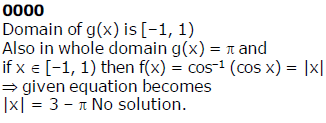*Answer can only contain numeric values
Part Test - 15 (JEE Advanced) - Question 58

The probability that x3 + ax2 + bx + c is divisible by x2+1. a,b,c,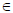{1, 2, 3,... k} is Pk. Find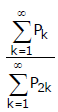Detailed Solution for Part Test - 15 (JEE Advanced) - Question 58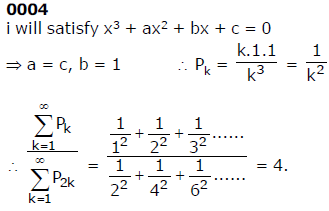*Answer can only contain numeric values
Part Test - 15 (JEE Advanced) - Question 59

If A1A2,....A2n is regular 2n side polygon. Find the ratio of number of obtuse triangles to the number of acute triangles formed by joining the vertices of the polygon

Detailed Solution for Part Test - 15 (JEE Advanced) - Question 59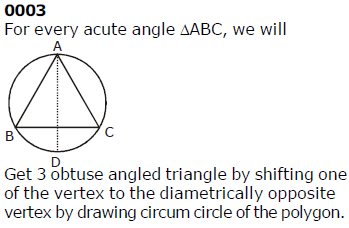*Answer can only contain numeric values
Part Test - 15 (JEE Advanced) - Question 60

f(x) and g(x) are polynomials of degree 3. g(α) =g’(α) = 0.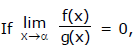then find the
number of different solutions of equation f(x) g’(x) + f’(x) g(x) = 0.

Detailed Solution for Part Test - 15 (JEE Advanced) - Question 60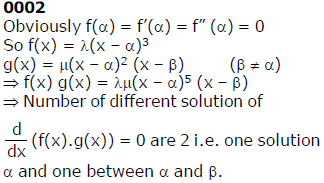## National Level Test Series for JEE Advanced 2020

3 videos|3 docs|40 tests
 Use Code STAYHOME200 and get INR 200 additional OFF Use Coupon Code